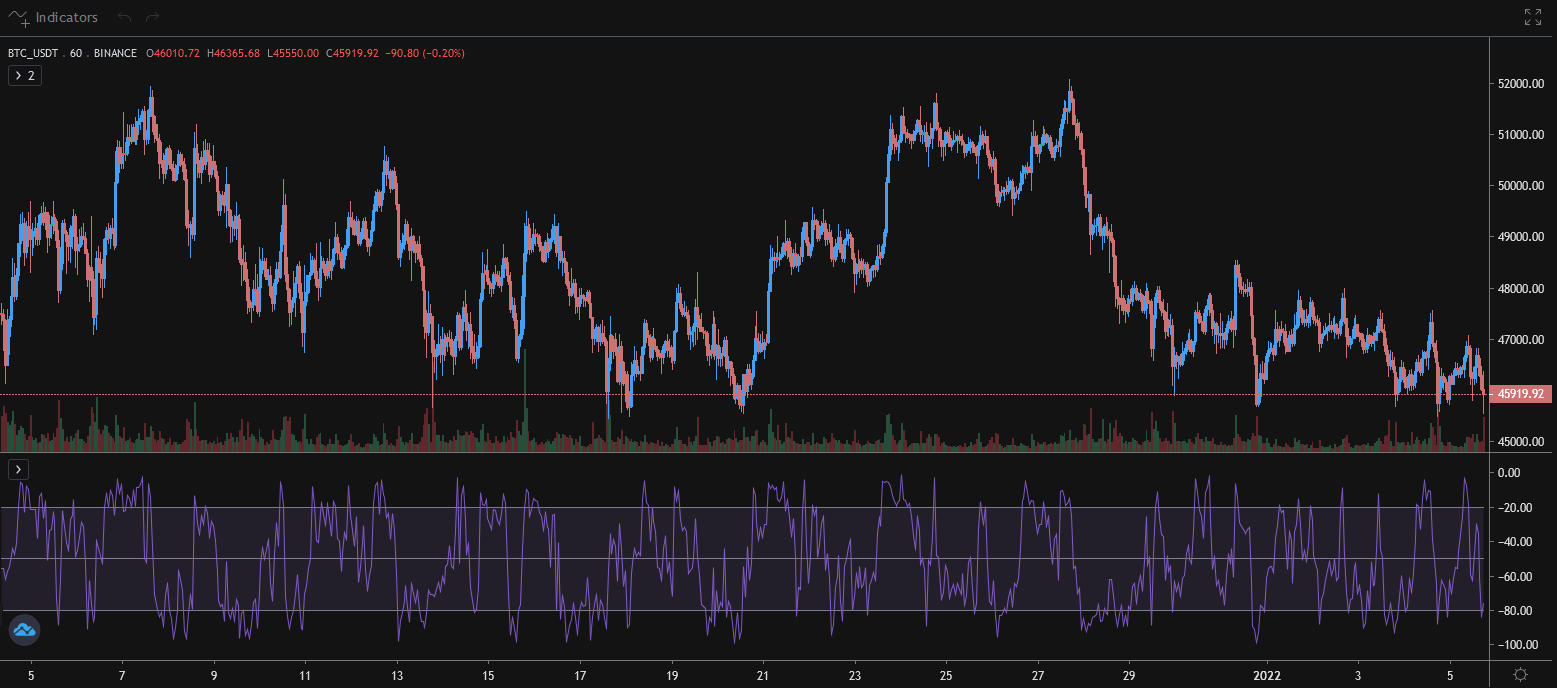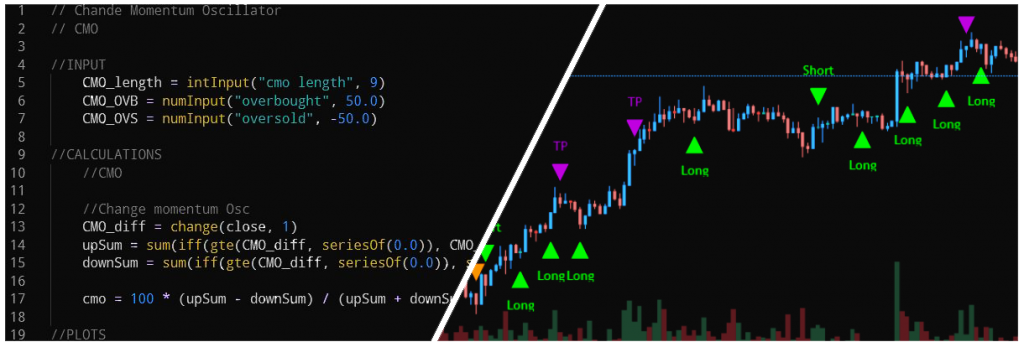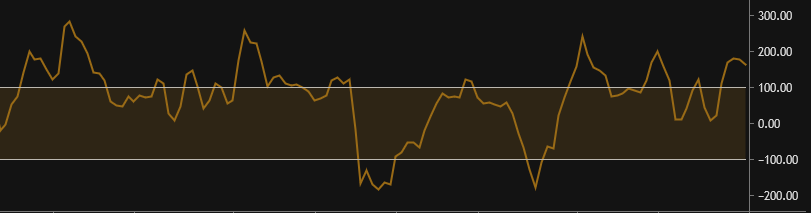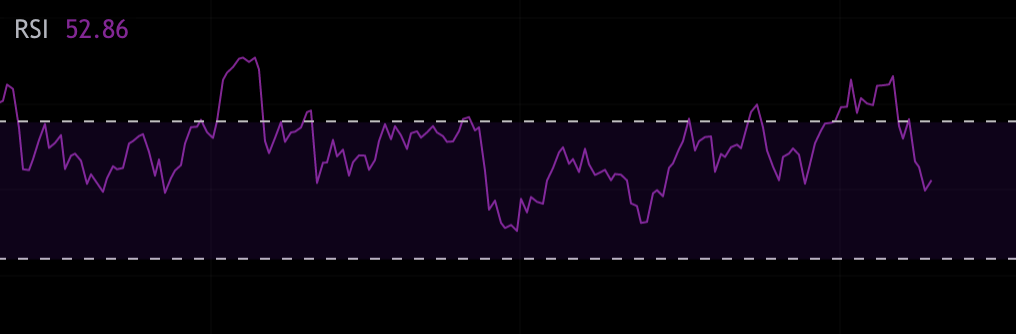## Price Action Series Chapter 3: Plot Support/ResistanceIn this chapter, we dive right into the rules for drawing support and resistance zones. We define a zone by taking into account: Swing highs and swing lows, meaning the highest and lowest points price reaches in a time period; Multiple touches and bounces off an area, the more the better; If the area you’re…

## What is the Williams %R indicator and how to use it on Tuned?We will look at what it does, how it’s used, and how it can be utilized on Tuned for algorithmic trading.

## What is the Momentum Indicator and how to use it on Tuned?In this article, we’ll be talking about the Momentum indicator.We’ll be exploring what it does, its common uses and how it can be applied to Tuned and algorithmic trading in general. Table of contents What is the Momentum Indicator? How is Momentum calculated? Common uses An example strategy using Momentum on Tuned Conclusion What is…

## The Importance of Diversification

Table of contents: What is diversification? Process of diversification on Tuned Understanding the risk to reward ratio of strategies and syndications Know your risk tolerance Choosing a mix of high and low risk strategies The monitoring and rebalancing process Conclusion What Is diversification? Diversification is the act of spreading your investments across various asset classes…

## Trading Strategies Supported By TunedWith Tuned, traders using Tuned Script or Pine Script Alpha can develop trading strategies, backtest them and run them live on their favorite exchanges. In this blog post, we’ll give you a quick run down on what strategy creators on Tuned are doing today. With your feedback, we aim to support all quantitative traders, so…

## What Is Tuned Script and How Is It Different From Pine Script?Table of contents: An alternative to Pine Script Data Flow Why two different languages? Example of Pine Script (RSI) Example of Tuned Script (RSI) Executing Orders Plotting – visualize a strategy An alternative to Pine Script Tuned Script is an in-house scripting language developed to write trading strategies. It is powered by Groovy with a…

## What is CCI and How to Use It in Tuned ScriptWhat is the Commodity Channel Index (CCI)? Key points about the CCI How to add the CCI to my script? How to use the CCI to generate buy and sell signals? What Is the Commodity Channel Index(CCI)? The CCI is a momentum-based oscillator used to help determine when an asset is reaching a condition of…

## What is MACD and How to Use It in Tuned ScriptWhat Is Moving Average Convergence Divergence (MACD)? Moving Average Convergence Divergence (MACD) is a trend-following momentum indicator that shows the relationship between two moving averages of an assets price. The MACD is calculated by subtracting the 26-period exponential moving average (EMA) from the 12-period EMA. The outcome of that calculation is the MACD line. A 9-period EMA of…

## What is RSI and How to Use It in Tuned ScriptWhat Is the Relative Strength Index (RSI)? The relative strength index (RSI) is a momentum indicator used in technical analysis that measures the magnitude of recent price changes to evaluate overbought or oversold conditions in the price of a stock or other asset. The RSI is displayed as an oscillator (a line graph that moves…